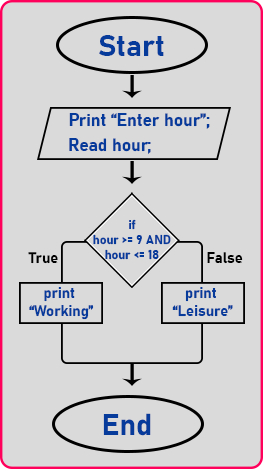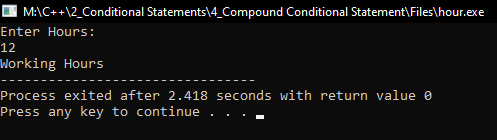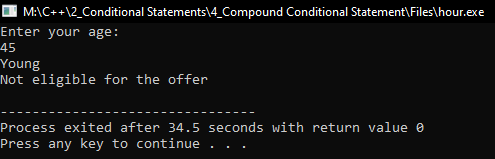# Compound Conditional Statements in C++

## Compound Conditional Statements in C++ with Examples

In this article, I am going to discuss Compound Conditional Statements in C++ with Examples. In the previous article, we have learned about Logical Operators that are used for writing compound conditional statements. We can combine more than one conditional statement by using logical ‘AND’ or ‘OR’ Operators. Let’s take an example and write a conditional statement and write the program on that.

##### Compound Conditional Statement:

Let us take an example of working hours and leisure hours. So, in the daytime, the hours start from ‘0’ to ‘23’ hours, total ‘24’ including zero. Now in this, from morning 9:00 to evening 6:00. These are taken as working hours. 6:00 of the evening will be taken as 18 hours in a 24-hour clock. People do a job between 9 to 18. So, the job hours are from 9 to 18 in a 24-hour clock.

Now we want to find out whether a given hour is a working hour or a leisure hour. So, if I say 10:00 then it’s a working hour. If I said 12:00, it’s working hour. If I say 17:00 then also it is working. So beyond 18, it’s not working hours. So, this is what I want to check.

##### Flowchart for finding working hours and leisure hoursSo, for finding working hours and leisure hours, I have a flowchart here, first we print ‘Enter hour’ and then we read the hour given by the user. Then if an hour is between 9 to 18 then it should print working hours otherwise it should print leisure. It means it’s not a working hour then how to frame a condition that hour should be within this range. So let us write a condition for that.

If (hour >= 9 && hour <= 18). So, condition looks like this.

In mathematics we write like: 9 <= hour <= 18. But in programming, we cannot write the things like this actually these are two different conditions and they are joined together. So, for both the condition we have to write hour, both the times. Now let us convert this into a program.

##### Working Hour Program Code in C++:
```#include <iostream>
using namespace std;

int main()
{
int hour;

cout << "Enter Hours:" << endl;
cin >> hour;

if (hour >= 9 && hour <= 18)
{
cout << "Working Hours";
}
else
{
cout << "Leisure Hours";
}

return 0;
}
```
###### Output:##### Age Validation Program Code in C++:
```#include <iostream>
using namespace std;
int main()
{
int age;

cout << "Enter your age: " << endl;
cin >> age;

if (age >= 12 && age <= 50)
{
cout << "Young" << endl;
}
else
{
cout << "Not Young" << endl;
}

if (age < 12 || age > 50)
{
cout << "Eligible for the offer" << endl;
}
else
{
cout << "Not eligible for the offer" << endl;
}

return 0;
}
```
###### Output:In the next article, I am going to discuss Nested If Conditional Statement in C++ with Examples. Here, in this article, I try to explain Compound Conditional Statements in C++ with Examples and I hope you enjoy this Compound Conditional Statements in C++ with Examples article.